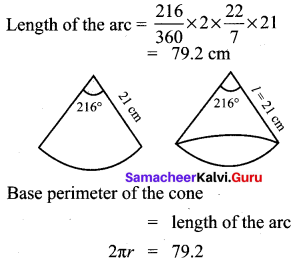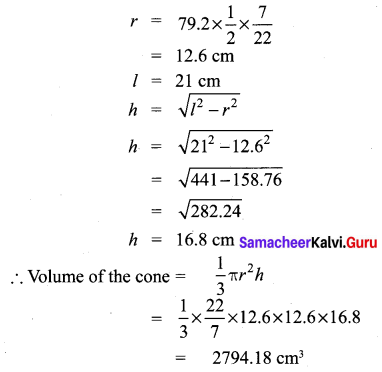# Samacheer Kalvi 10th Maths Solutions Chapter 7 Mensuration Unit Exercise 7

## Tamilnadu Samacheer Kalvi 10th Maths Solutions Chapter 7 Mensuration Unit Exercise 7

Question 1.
The barrel of a fountain-pen cylindrical in shape, is 7 cm long and 5 mm in diameter. A full barrel of ink in the pen will be used for writing 330 words on an average. How many words can be written using a bottle of ink containing one fifth of a litre?
Solution: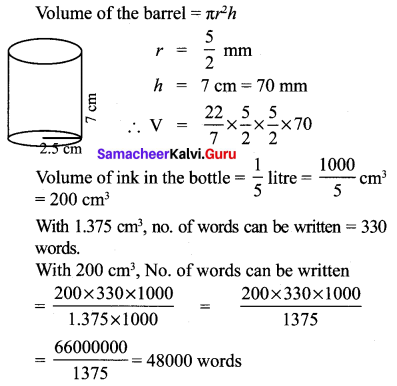Question 2.
A hemi-spherical tank of radius 1.75 m is full of water. It is connected with a pipe which empties the tank at the rate of 7 litre per second. How much time will it take to empty the tank completely?
Solution:
Suppose the pipe takes x seconds to empty the tank. Then, volume of the water that flows out of the tank in x seconds = Volume of the hemispherical tank.
Volume of the water that flows out of the tank in x seconds.
= Volume of hemispherical shell of radius 175 cm.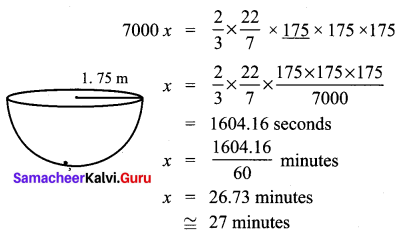Question 3.
Find the maximum volume of a cone that can be carved out of a solid hemisphere of radius r units.
Solution:
Radius of the base of cone = Radius of the hemisphere = r
Height of the cone = Radius of the hemisphere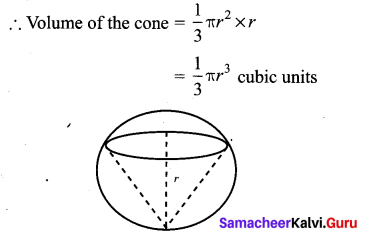Question 4.
An oil funnel of tin sheet consists of a cylindrical portion 10 cm long attached to a frustum of a cone. If the total height is 22 cm, the diameter of the cylindrical portion be 8cm and the diameter of the top of the funnel be 18 cm, then find the area of the tin sheet required to make the funnel.
Solution:
Slant height of the frustum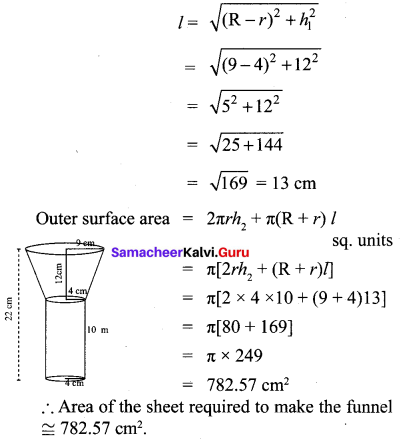Question 5.
Find the number of coins, 1.5 cm in diameter and 2 mm thick, to be melted to form a right circular cylinder of height 10 cm and diameter 4.5 cm.
Solution:
No. of coins required .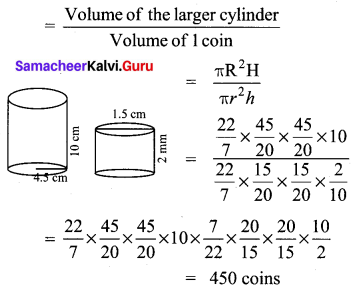Question 6.
A hollow metallic cylinder whose external radius is 4.3 cm and internal radius is 1.1 cm
and whole length is 4 cm is melted and recast into a solid cylinder of 12 cm long. Find the diameter of solid cylinder.
Solution:
Volume of the solid cylinder = Volume of the hollow cylinder melted.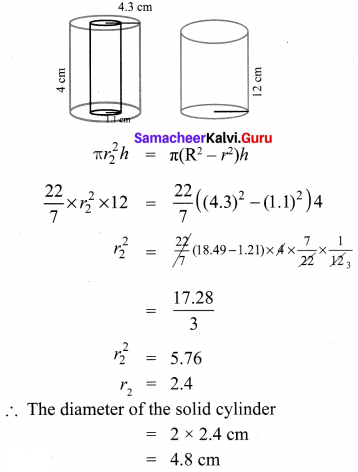Question 7.
The slant height of a frustum of a cone is 4 m and the perimeter of circular ends are 18 m and 16 m. Find the cost of painting its curved surface area at ₹ 100 per sq. m.
Solution: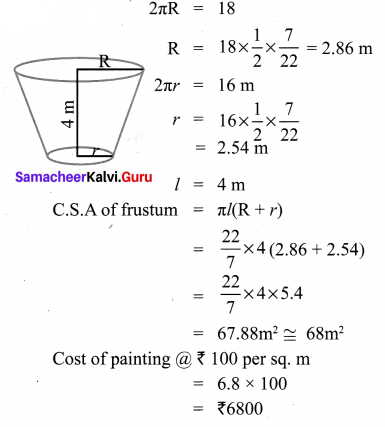Question 8.
A hemi-spherical hollow bowl has material of volume $$\frac{436 \pi}{3}$$ cubic cm. Its external diameter is 14 cm. Find its thickness.
Solution: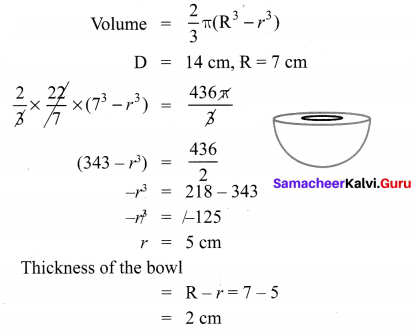Question 9.
The volume of a cone is $$1005 \frac{5}{7}$$ cu. cm. The area of its base is $$201 \frac{1}{7}$$ sq. cm. Find the slant height of the cone.
Solution: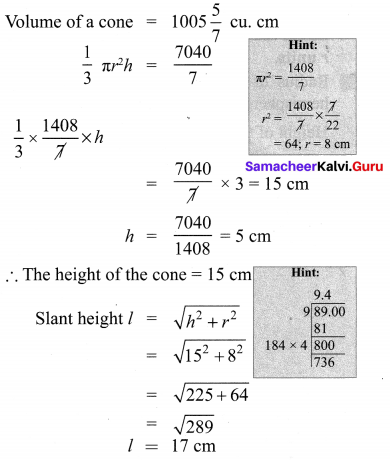Question 10.
A metallic sheet in the form of a sector of T a circle of radius 21 cm has central angle of 216°. The sector is made into a cone by bringing the bounding radii together. Find the volume of the cone formed.
Solution: